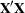Displayed Output

PROC VARCOMP displays the following items:

• Class Level Information for verifying the levels in your data

• Number of observations read from the data set and number of observations used in the analysis

• for METHOD=TYPE1, an analysis-of-variance table with Source, DF, Type I Sum of Squares, Type I Mean Square, and Expected Mean Square, and a table of Type I variance component estimates

• for METHOD=MIVQUE0, the SSQ Matrix containing sums of squares of partitions of thecrossproducts matrix adjusted for the fixed effects

• for METHOD=ML and METHOD=REML, the iteration history, including the objective function, a table of variance component estimates, and the estimated Asymptotic Covariance Matrix of the variance components

• for METHOD=GRR, an analysis-of-variance table with Source, DF, GRR Sum of Squares, GRR Mean Square, and Expected Mean Square, and a table of GRR parameter estimates. If the CL option is specified, confidence limits for each parameter estimate will also be displayed.